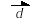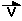### Kinematics - The description of Motion

#### There are two types of motion:

• Constant Motion (no acceleration - constant speed)
• Accelerated Motion (motion at changing speed)

Constant Motion obeys two conditions

1. must be in a straight line
2. must be at constant speed

Accelerated Motion

1. does not have to be in a straight line
2. speed changes over time intervals

#### Distance, Displacement, Speed, Velocity

Displacement is defined as the distance traveled in one direction.

This is the symbol for displacement• Displacement is  a vector quantity.
Review the concepts associated with Vectors

The unit of displacement is the meter (m).  The symbol for distance is d.

Velocity is the speed an object travels in one particular direction.  The symbol for speed v and the
units for speed are " meters per second" or (m/s).

is the symbol for velocity.The symbol for speed is   V.

To calculate average speed (or the magnitude of the average velocity) we use the simplified equationExample:

If you walk your dog at 30 cm/s.  How far will you have traveled in 20 minutes?

Given:  v = 30 cm/s = 0.30 m/s
t = 20 min. = 20 min X 60 s/min = 1200 s

Find: d

Solution:   Use the equation v = d/t

then rearrange it to solve for d
d = v X t

Therefore    d = 0.30 m/s  X 1200 s  = 360 m

Therefore you would have walked a distance of 360 m

Acceleration

Acceleration is the rate at which speed increases or decreases.  When an object slows down we say it
is decelerating. Deceleration is negative acceleration.  The symbol for acceleration is a.  The units for acceleration are m/s2     The equation for acceleration is  a = v/t.

Exercises

1.  Use the equation for speed to complete the following table

 Distance (m) Time (s) Speed (m/s) 12 6 0.02 35 300 20

2. Change the number 3 459 875 in scientific notation

3. A subway train traveling at 175 km/h takes 25 minutes to go between the Royal York and the Dufferin Stations.
What is the distance between these stations.

4. A cart is attached to a 5 Hz ticker tape timer.
The timer produces a series of evenly spaced dots when the cart is pushed gently on a smooth table.

An exact image of the tape is shown below.

.    .    .    .    .    .    .    .    .    .    .    .    .    .    .    .    .    .    .    .    .    .    .    .    .    .    .    .    .    .    .

a) Plot a speed time graph using the tape and calculate the slope of the line.

b) What type of motion is displayed by the tape?  Why?

c) If the cart was allowed to stop on its own (even after the tape ran out) how far did it go approximately after 12 s?

d) What was the average speed of the cart during the 12 s?

e) What does the slope of the line indicate?

5. Give an example of uniform motion.

6. Give an example of non-uniform motion.